# Python <算法思想集结>之抽丝剥茧聊动态规划

## 1. 概述

`动态规划`算法应用非常之广泛。

• 重叠子问题。
• 最优子结构。
• 状态转移。

`动态规划``分治算法`有一个相似之处。

• 分治算法的每一个子问题具有完全独立性，只会被计算一次。

`二分查找`是典型的`分治算法`实现，其子问题是把数列缩小后再二分查找，每一个子问题只会被计算一次。

• 动态规划经分解得到的子问题往往不是互相独立的，有些子问题会被重复计算多次，这便是`重叠子问题`

• 同一个子问题被计算多次，完全是没有必要的，可以缓存已经计算过的子问题，再次需要子问题结果时只需要从缓存中获取便可。这便是动态规划中的典型操作，优化重叠子问题，通过`空间换时间`的优化手段提高性能。

`重叠子问题`并不是动态规划的专利，重叠子问题是一个很普见的现象。

## 2. 流程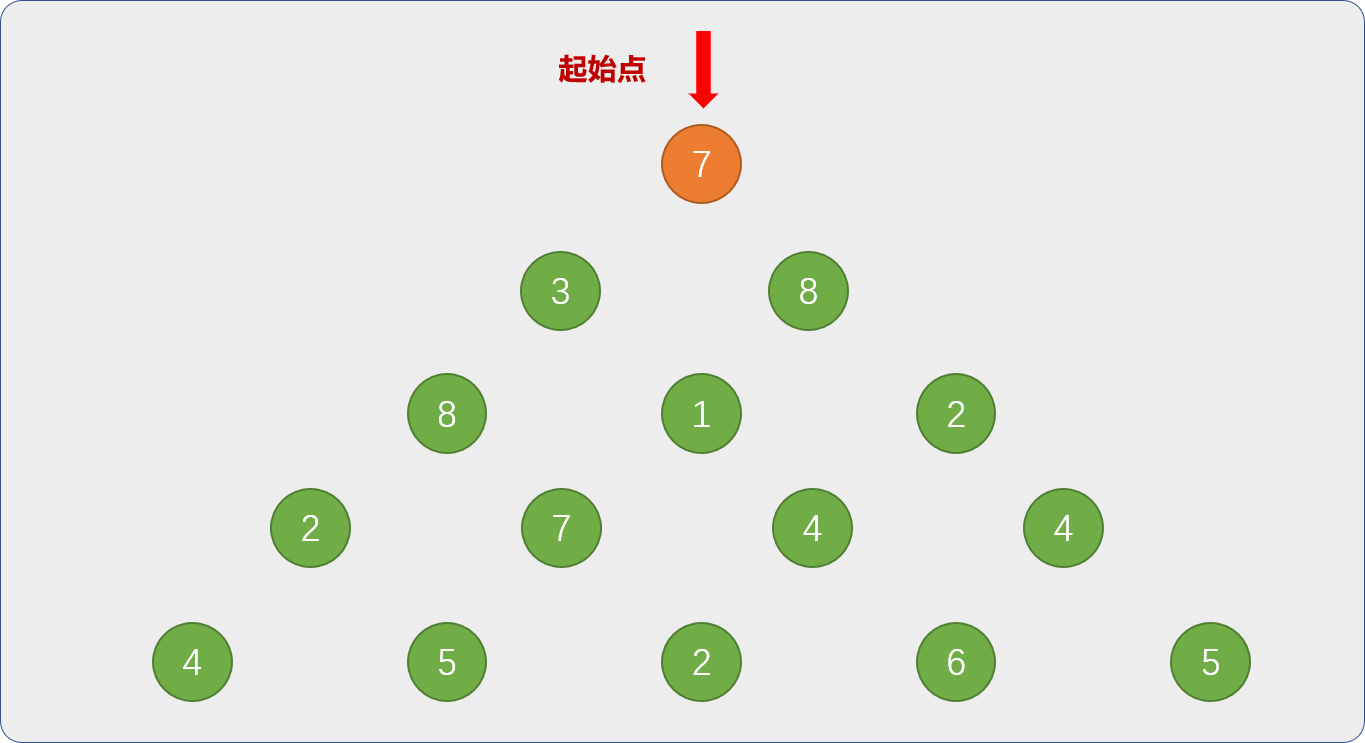## 2.1 是否存在子问题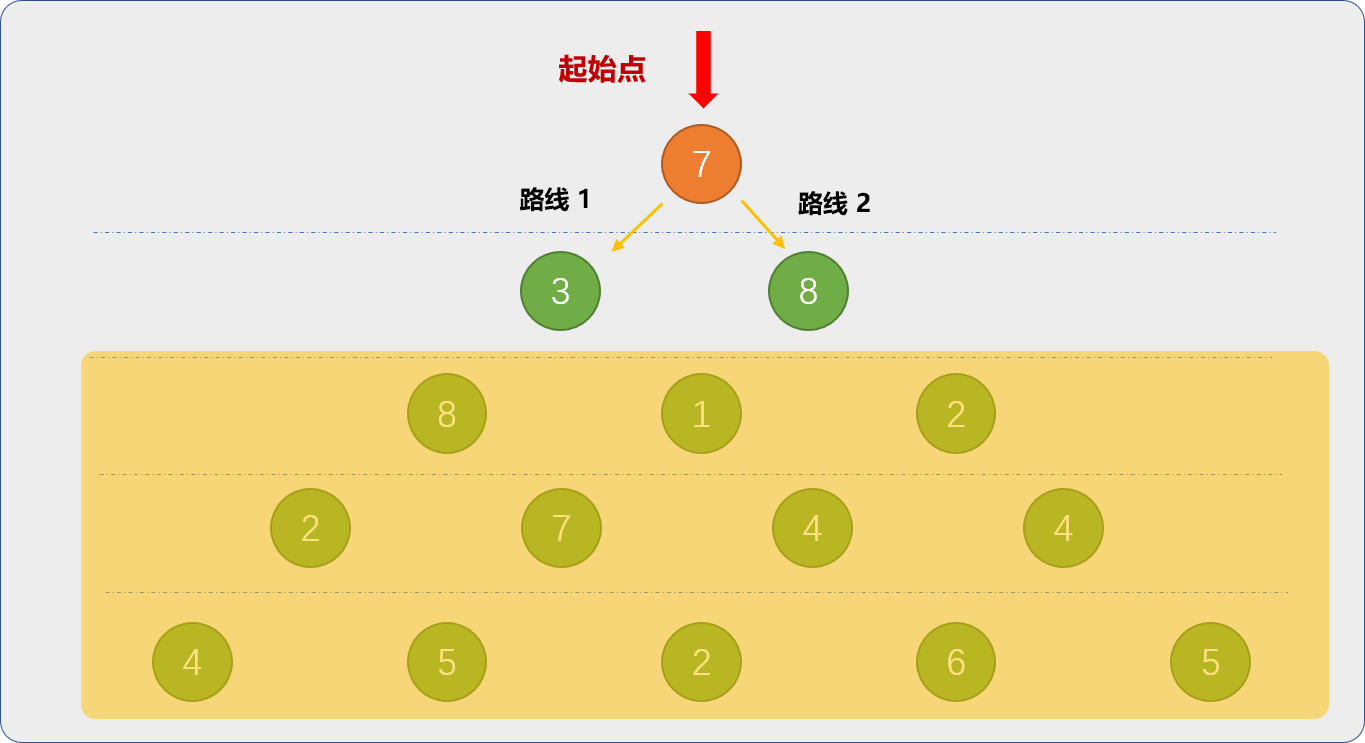• 先分别求解`路线 1``路线 2`上的最大值。`路线 1`的最大值为 `3`,`路线 2`上的最大值是`8`

• 然后求解出`路线 1``路线 2`两者之间的最大值 `8`。 把求得的结果和出发点的数字 `7` 相加，`7+8=15` 就是最后答案。

只有 `2` 行时，兔子能获得的最多萝卜数为 `15`，肉眼便能看的出来。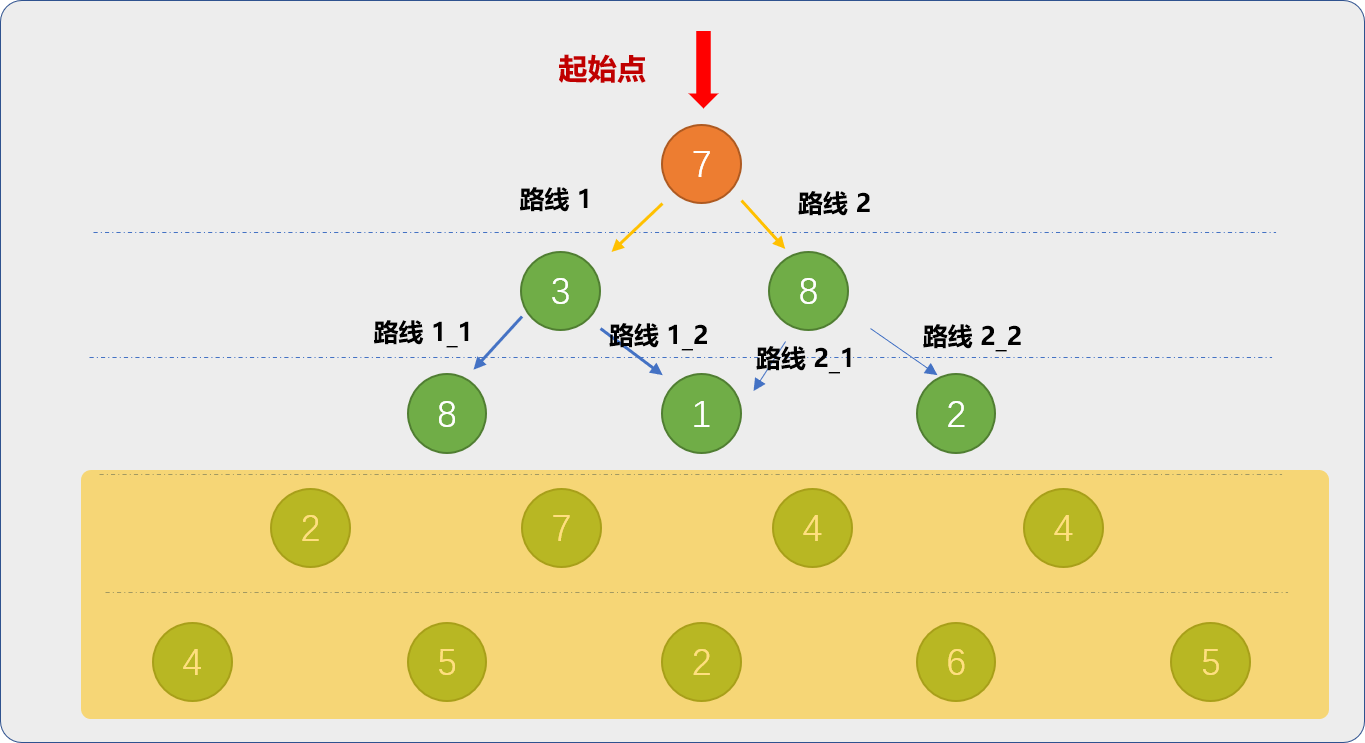• 路线 `1` 的子问题有 `2`个，`路线 1_1``路线1_2`。求解 `2` 个子问题的最大值后，再在 `2 `个子问题中选择最大值`8`，最后路线 `1`的最大值为`3+8=11`
• 路线 `2` 的子问题有 `2`个，`路线 2_1``路线2_2`。求解 `2` 个子问题的最大值后，再在 `2 `个子问题中选择最大值`2`，最后路线 `2`的最大值为`8+2=10`

• 使用`二维数列表`保存三角形数列中的所有数据。`a=[,[3,8],[8,1,2],[2,7,4,4],[4,5,2,6,5]]`

• 原始问题为 `f(0，0)`从数列的`(0,0)`出发，向左下角和右下角前行，一直找到此路径上的数字相加为最大。

`f(0,0)`表示以第 `1` 行的第 `1` 列数字为起始点。

• 分解原始问题 `f(0,0)=a(0,0)+max(f(1,0)+f(1,1))`

• 因为每一个子问题又可以分解，让表达式更通用 `f(i,j)=a(i,j)+max(f(i+1,j)+f(i+1,j+1))`

`(i+1,j)`表示 `(i,j)`的左下角，`(i+1,j+1)`表示 `(i,j)`的右下角，

``````# 已经数列
nums = [, [3, 8], [8, 1, 2], [2, 7, 4, 4], [4, 5, 2, 6, 5]]
# 递归函数
def get_max_lb(i, j):
if i == len(nums) - 1:
# 递归出口
return nums[i][j]
# 分解子问题
return nums[i][j] + max(get_max_lb(i + 1, j), get_max_lb(i + 1, j + 1))
# 测试
res = get_max_lb(0, 0)
print(res)
'''

30
'''
``````

## 2.2 是否存在重叠子问题

``````import random
nums = []
# 递归函数
def get_max_lb(i, j):
if i == len(nums) - 1:
return nums[i][j]
return nums[i][j] + max(get_max_lb(i + 1, j), get_max_lb(i + 1, j + 1))
# 构建 100 行的二维列表
for i in range(100):
nums.append([])
for j in range(i + 1):
nums[i].append(random.randint(1, 100))

res = get_max_lb(0, 0)
print(res)
``````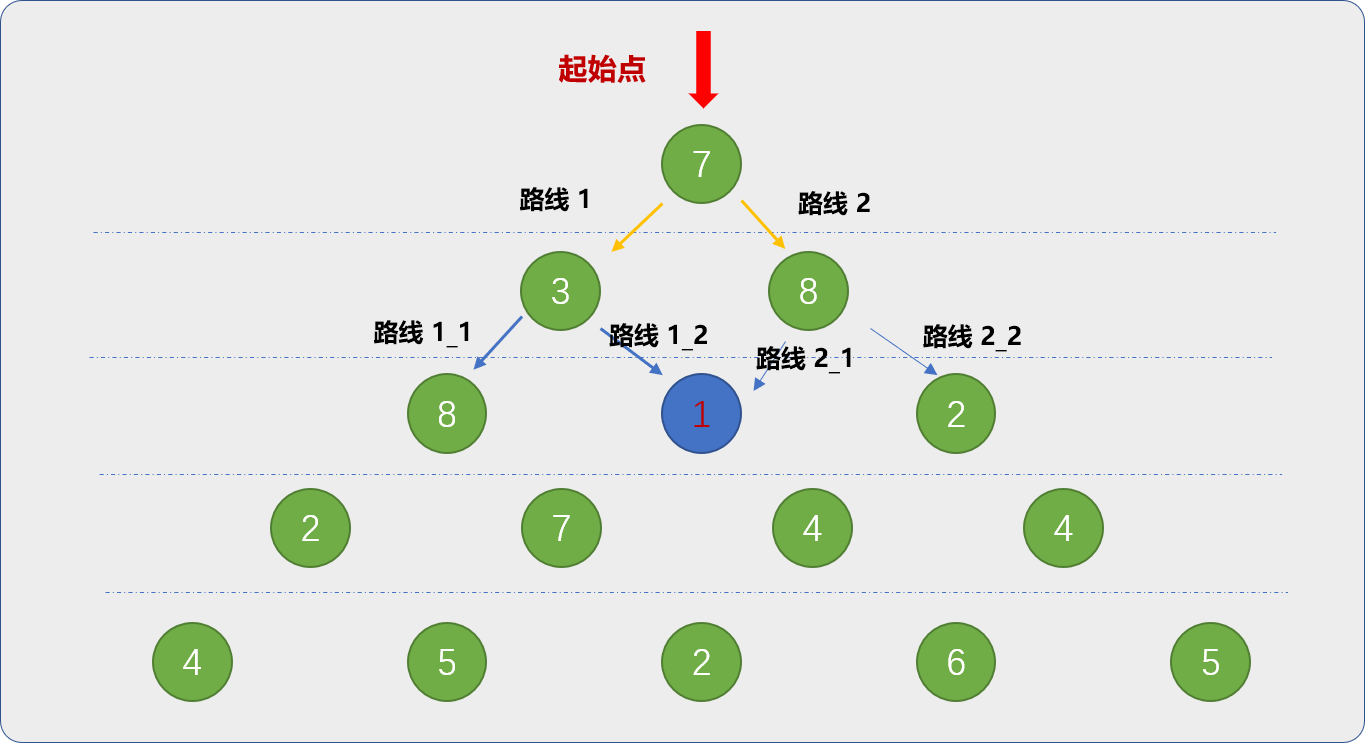`路线1_2``路线2_1`的起点都是从同一个地方（蓝色标注的位置）出发。显然，从数字 `1`（蓝色标注的数字）出发的这条路径会被计算 `2` 次。在上图中被重复计算的子路径可不止一条。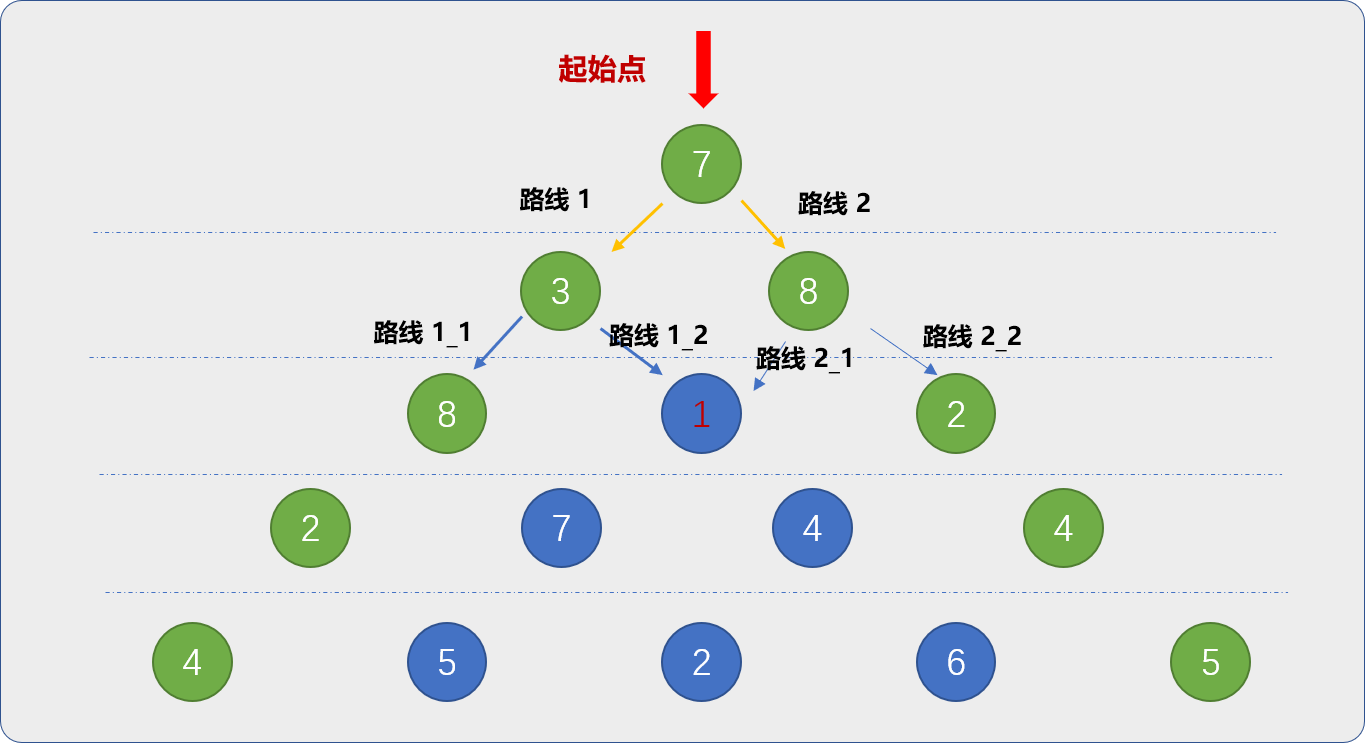``````import random
def get_max_lb(i, j):
if i == len(nums) - 1:
return nums[i][j]
left_max = None
right_max = None
if (i + 1, j) in dic.keys():
# 检查缓存中是否存在子问题的结果
left_max = dic[i + 1, j]
else:
# 缓存中没有，才递归求解
left_max = get_max_lb(i + 1, j)
# 求解后的结果缓存起来
dic[(i + 1, j)] = left_max
if (i + 1, j + 1) in dic.keys():
right_max = dic[i + 1, j + 1]
else:
right_max = get_max_lb(i + 1, j + 1)
dic[(i + 1, j + 1)] = right_max
return nums[i][j] + max(left_max, right_max)

# 已经数列
nums = []
# 缓存器
dic = {}
for i in range(100):
nums.append([])
for j in range(i + 1):
nums[i].append(random.randint(1, 100))
# 递归调用
res = get_max_lb(0, 0)
print(res)
``````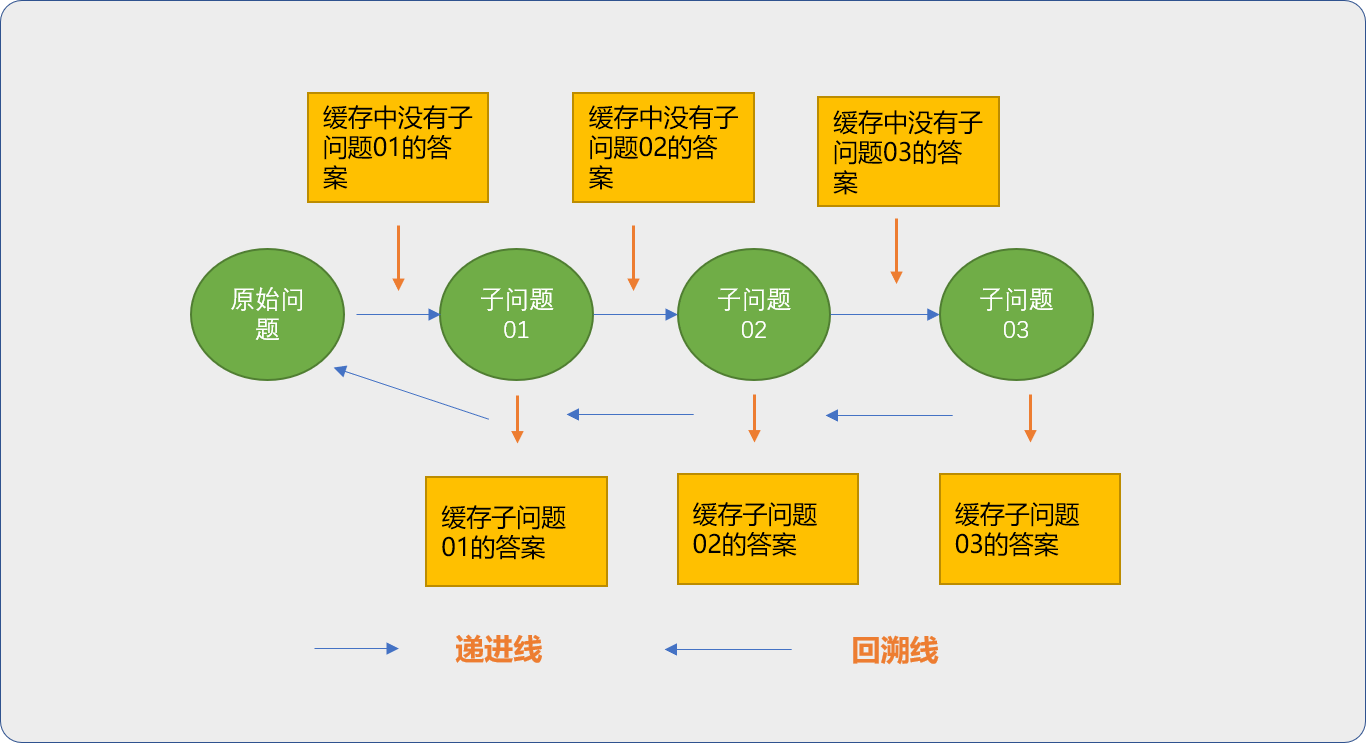## 2.3 状态转移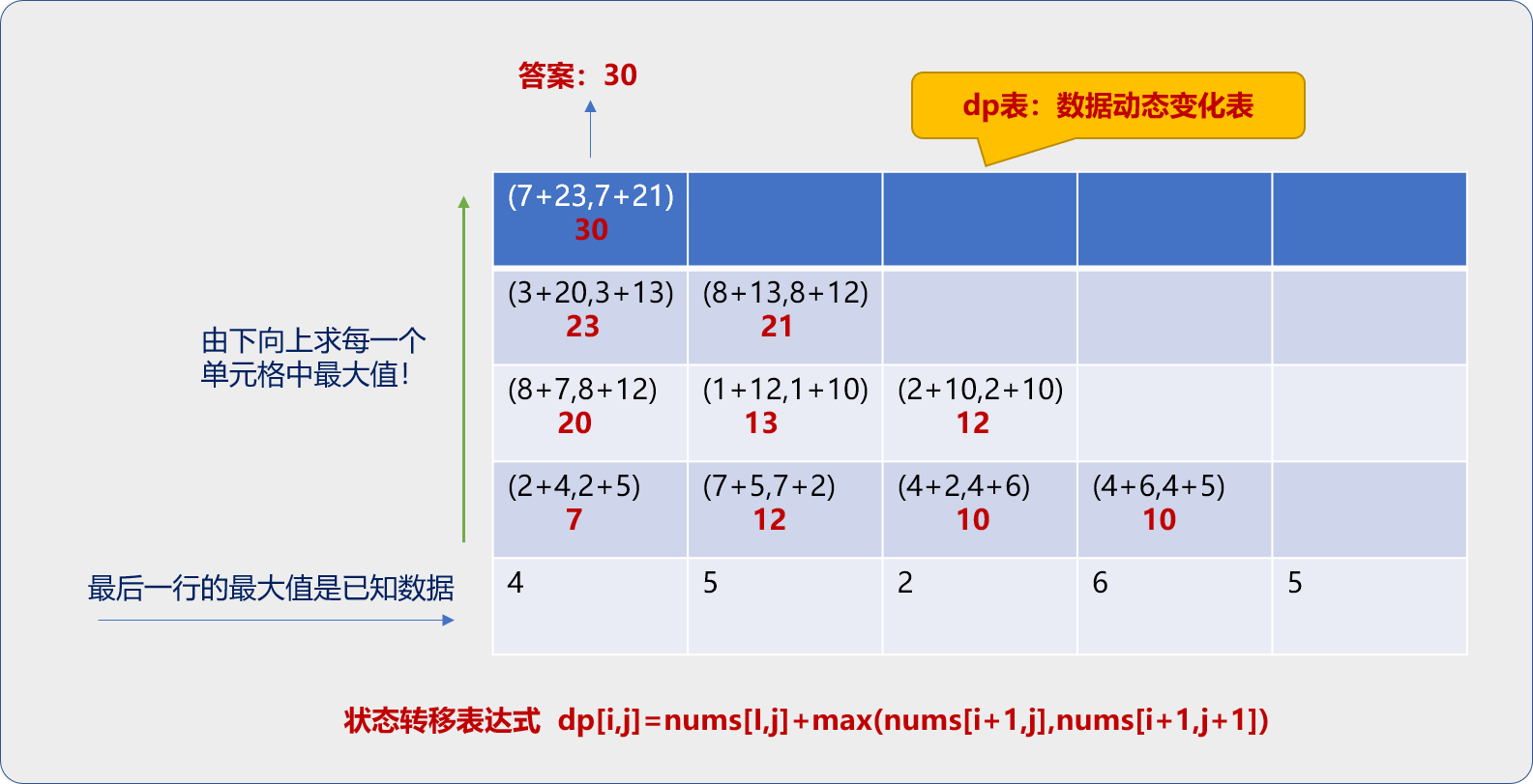``````nums = [, [3, 8], [8, 1, 2], [2, 7, 4, 4], [4, 5, 2, 6, 5]]

# dp列表
dp = []
idx = 0
# 从最后一行开始
for i in range(len(nums) - 1, -1, -1):
dp.append([])
for j in range(len(nums[i])):
if i == len(nums) - 1:
# 最后一行缓存于状态转移表中
dp[idx].append(nums[i][j])
else:
dp[idx].append(nums[i][j] + max(dp[idx - 1][j], dp[idx - 1][j + 1]))
idx += 1

print(dp)
'''

[[4, 5, 2, 6, 5], [7, 12, 10, 10], [20, 13, 12], [23, 21], ]
'''
``````

``````nums = [, [3, 8], [8, 1, 2], [2, 7, 4, 4], [4, 5, 2, 6, 5]]

# dp表
dp = []
# 临时表
tmp = []
# 从最后一行开始
for i in range(len(nums) - 1, -1, -1):
# 把上一步得到的状态数据提出来
tmp = dp.copy()
# 清除 dp 表中原来的数据，准备保存最新的状态数据
dp.clear()
for j in range(len(nums[i])):
if i == len(nums) - 1:
# 最后一行缓存于状态转移表中
dp.append(nums[i][j])
else:
dp.append(nums[i][j] + max(tmp[j], tmp[j + 1]))

print(dp)
'''


'''
``````